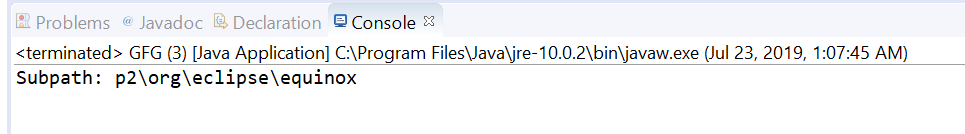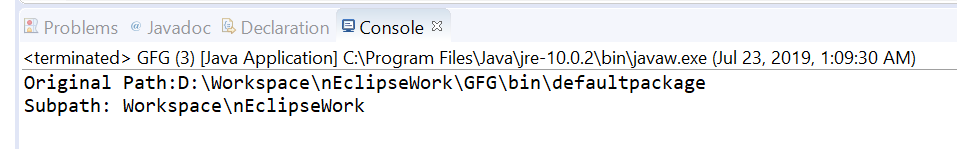Related Articles
Path subpath() method in Java with Examples
• Last Updated : 28 Jul, 2019

The subpath(int beginIndex, int endIndex) method of java.nio.file.Path used to return a relative Path that is a subsequence of the name elements of this path. We will pass begin and end indexes to construct a subpath. The beginIndex and endIndex parameters specify the subsequence of name elements. The name element closest to the root in the directory hierarchy is index 0 and name element that is farthest from the root has index count-1. The returned subpath object has the name elements that begin at beginIndex and extend to the element at index endIndex-1.

Syntax:

```Path subpath(int beginIndex,
int endIndex)
```

Parameters: This method accepts a two parameters:

• beginIndex which is the index of the first element, inclusive and
• endIndex which is the the index of the last element, exclusive.

Return value: This method returns a new Path object that is a subsequence of the name elements in this Path.

Exception: This method throws IllegalArgumentException if beginIndex is negative, or greater than or equal to the number of elements. If endIndex is less than or equal to beginIndex, or larger than the number of elements.

Below programs illustrate subpath() method:
Program 1:

 `// Java program to demonstrate ` `// java.nio.file.Path.subpath() method ` ` `  `import` `java.nio.file.Path; ` `import` `java.nio.file.Paths; ` `public` `class` `GFG { ` `    ``public` `static` `void` `main(String[] args) ` `    ``{ ` ` `  `        ``// create an object of Path ` `        ``Path path ` `            ``= Paths.get(``"D:\\eclipse\\p2"` `                        ``+ ``"\\org\\eclipse\\equinox\\p2\\core"` `                        ``+ ``"\\cache\\binary"``); ` ` `  `        ``// call subPath() to create a subPath which ` `        ``// begin at index 1 and ends at index 5 ` `        ``Path subPath = path.subpath(``1``, ``5``); ` ` `  `        ``// print result ` `        ``System.out.println(``"Subpath: "` `                           ``+ subPath); ` `    ``} ` `} `

Output:Program 2:

 `// Java program to demonstrate ` `// java.nio.file.Path.subpath() method ` ` `  `import` `java.nio.file.Path; ` `import` `java.nio.file.Paths; ` `public` `class` `GFG { ` `    ``public` `static` `void` `main(String[] args) ` `    ``{ ` ` `  `        ``// create an object of Path ` `        ``Path path ` `            ``= Paths.get(``"D:\\Workspace"` `                        ``+ ``"\\nEclipseWork"` `                        ``+ ``"\\GFG\\bin\\defaultpackage"``); ` ` `  `        ``System.out.println(``"Original Path:"` `                           ``+ path); ` ` `  `        ``// call subPath() to create a subPath which ` `        ``// begin at index 0 and ends at index 2 ` `        ``Path subPath = path.subpath(``0``, ``2``); ` ` `  `        ``// print result ` `        ``System.out.println(``"Subpath: "` `                           ``+ subPath); ` `    ``} ` `} `

Output:Attention reader! Don’t stop learning now. Get hold of all the important Java Foundation and Collections concepts with the Fundamentals of Java and Java Collections Course at a student-friendly price and become industry ready.

My Personal Notes arrow_drop_up
Recommended Articles
Page :